Courses

Common: Collector Configuration Notes | Study Analog Electronics - Electrical Engineering (EE)

Electrical Engineering (EE): Common: Collector Configuration Notes | Study Analog Electronics - Electrical Engineering (EE)

The document Common: Collector Configuration Notes | Study Analog Electronics - Electrical Engineering (EE) is a part of the Electrical Engineering (EE) Course Analog Electronics.
All you need of Electrical Engineering (EE) at this link: Electrical Engineering (EE)

COMMON – COLLECTOR CONFIGURATION

Also called emitter-follower (EF). It is called common-emitter configuration since both the signal source and the load share the collector terminal as a common connection point. The output voltage is obtained at the emitter terminal. The input characteristics of common-collector configuration is similar with common-emitter configuration.Common-collector circuit configuration is provided with the load resistor connected from the emitter to the ground. It is used primarily for impedance-matching purpose since it has high input impedance and low output impedance.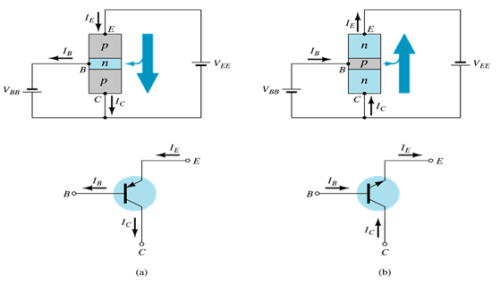For the common-collector configuration, the output characteristics are a plot of Ivs VCE for a range of values of IB.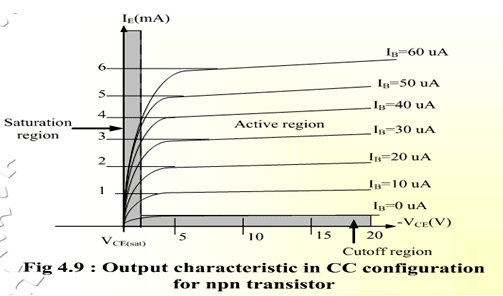Limits of opearation:

Many BJT transistors are used as an amplifier. Thus it is important to notice the limits of operations. At least 3 maximum values are mentioned in the data sheet.

They are:

a) Maximum power dissipation at collector: PCmax or PD.

b) Maximum collector-emitter voltage: VCEmax sometimes named as VBR(CEO) or VCEO.

c) Maximum collector current: ICmax.

There are few rules which need to be followed for BJT transistor to be used as an amplifier. The rules are: The transistor must be operated in active region!

IC < ICmax

PC < PCmax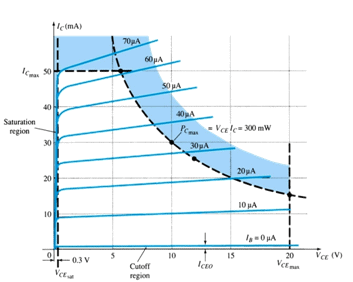Note:          VCE is at maximum and IC is at minimum (ICmax=ICEO) in the cutoff region. IC is at maximum and VCE is at minimum (VCEmax = VCESAT = VCEO) in the saturation region. The transistor operates in the active region between saturation and cutoff.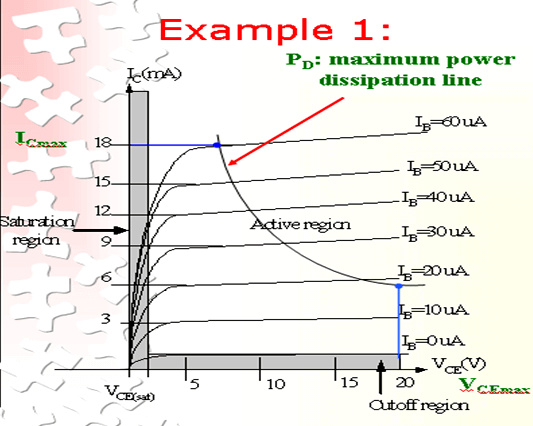Refer to the fig. Example; A derating factor of 2mW/°C indicates the power dissipation is reduced 2mW each degree centigrade increase of temperature.

Step1:

The maximum collector power dissipation,

PD=ICMAX x VCEmax= 18m x 20 = 360 mW

Step 2:

At any point on the characteristics the product of and must be equal to 360 mW.

Ex. 1. If choose ICmax= 5 mA, substitute into the (1), we get

VCEmaxICmax= 360 mW

VCEmax(5 m)=360/5=7.2 V

Ex.2. If choose VCEmax=18 V, substitute into (1), we get

VCEmaxICmax= 360 mW

(10) ICMAX=360m/18=20 mA

Derating PDmax

PDMAX is usually specified at 25°C.

The higher temperature goes, the less is PDMAX

Example;A derating factor of 2mW/°C indicates the power dissipation is reduced 2mW each degree centigrade increase of temperature.

The document Common: Collector Configuration Notes | Study Analog Electronics - Electrical Engineering (EE) is a part of the Electrical Engineering (EE) Course Analog Electronics.
All you need of Electrical Engineering (EE) at this link: Electrical Engineering (EE)Use Code STAYHOME200 and get INR 200 additional OFF Use Coupon Code

Top Courses for Electrical Engineering (EE)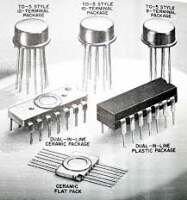Analog Electronics

11 videos|56 docs|97 tests

Top Courses for Electrical Engineering (EE)Track your progress, build streaks, highlight & save important lessons and more!

,

,

,

,

,

,

,

,

,

,

,

,

,

,

,

,

,

,

,

,

,

;